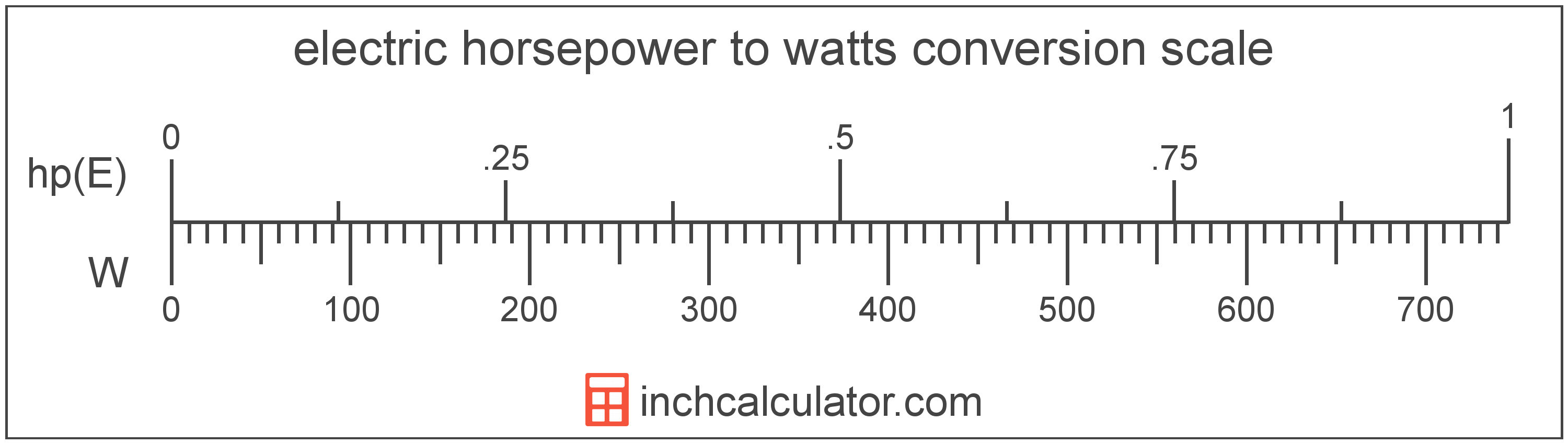# Convert Watts to Electric Horsepower

Enter the power in watts below to get the value converted to electric horsepower.

Results in Electric Horsepower:1 W = 0.00134 hp(E)

## How to Convert Watts to Electric HorsepowerTo convert a watt measurement to a electric horsepower measurement, multiply the power by the conversion ratio. One watt is equal to 0.00134 electric horsepower, so use this simple formula to convert:

electric horsepower = watts × 0.00134

The power in electric horsepower is equal to the watts multiplied by 0.00134.

For example, here's how to convert 5 watts to electric horsepower using the formula above.
5 W = (5 × 0.00134) = 0.006702 hp(E)

Watts and electric horsepower are both units used to measure power. Keep reading to learn more about each unit of measure.

## Watts

One watt is the power equal to the rate of energy of one joule per second in an electrical circuit. One watt is also equal to the power produced in a circuit with a voltage potential of one volt with a current of one amp.

The watt is the SI derived unit for power in the metric system. Watts can be abbreviated as W, for example 1 watt can be written as 1 W.

Watt's Law states the relationship between power, current, and voltage. Using Watt's Law, it's possible to express the power in watts as an expression using current and voltage.

PW = IA × VV

The power in watts is equal to the current in amperes times the potential difference in volts.

Combining this with Ohm's Law, it's also possible to express the power in watts using resistance in addition to volts and amps.

## Electric Horsepower

Electric horsepower is used to measure the output of electric motors and other electrical machines in the US. One electric horsepower is equal to exactly 746 watts.

The electric horsepower is a US customary and imperial unit of power. Electric horsepower can be abbreviated as hp(E), for example 1 electric horsepower can be written as 1 hp(E).

## Watt Measurements and Equivalent Electric Horsepower Conversions

Common watt values converted to the equivalent electric horsepower value
Watts Electric Horsepower
1 W 0.00134 hp(E)
2 W 0.002681 hp(E)
3 W 0.004021 hp(E)
4 W 0.005362 hp(E)
5 W 0.006702 hp(E)
6 W 0.008043 hp(E)
7 W 0.009383 hp(E)
8 W 0.010724 hp(E)
9 W 0.012064 hp(E)
10 W 0.013405 hp(E)
11 W 0.014745 hp(E)
12 W 0.016086 hp(E)
13 W 0.017426 hp(E)
14 W 0.018767 hp(E)
15 W 0.020107 hp(E)
16 W 0.021448 hp(E)
17 W 0.022788 hp(E)
18 W 0.024129 hp(E)
19 W 0.025469 hp(E)
20 W 0.02681 hp(E)
21 W 0.02815 hp(E)
22 W 0.029491 hp(E)
23 W 0.030831 hp(E)
24 W 0.032172 hp(E)
25 W 0.033512 hp(E)
26 W 0.034853 hp(E)
27 W 0.036193 hp(E)
28 W 0.037534 hp(E)
29 W 0.038874 hp(E)
30 W 0.040214 hp(E)
31 W 0.041555 hp(E)
32 W 0.042895 hp(E)
33 W 0.044236 hp(E)
34 W 0.045576 hp(E)
35 W 0.046917 hp(E)
36 W 0.048257 hp(E)
37 W 0.049598 hp(E)
38 W 0.050938 hp(E)
39 W 0.052279 hp(E)
40 W 0.053619 hp(E)

## More Watt Power Conversions

SI Units
Convert to Microwatts
1 W is equal to 1,000,000 microwatts
Convert to Milliwatts
1 W is equal to 1,000 milliwatts
Convert to Kilowatts
1 W is equal to 0.001 kilowatts
Convert to Megawatts
1 W is equal to 1.0E-6 megawatts
US Customary & Imperial Units
Convert to Mechanical Horsepower
1 W is equal to 0.001341 mechanical horsepower
Convert to Btu Per Hour
1 W is equal to 3.412142 btu per hour
Other Units
Convert to Kilocalories Per Hour
1 W is equal to 0.860421 kilocalories per hour